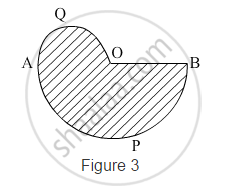Share

# In Fig. 3, APB and AQO are semicircles, and AO = OB. If the perimeter of the figure is 40 cm, find the area of the shaded region - CBSE Class 10 - Mathematics

ConceptProblems Based on Areas and Perimeter Or Circumference of Circle, Sector and Segment of a Circle

#### Question

In Fig. 3, APB and AQO are semicircles, and AO = OB. If the perimeter of the figure is 40 cm, find the area of the shaded region [Use pi=22/7]#### Solution

Let the radius of the semi-circle APB be r

⇒ The radius of the semi-circle AQO =r/2

Now,
Perimeter of the given figure = Length of arc AQO + Length of arc APB + OB

=pixxr/2+pixxr+r

=r(3/2pi+1)

=r(3/2xx22/7+1)

= r((33+7)/7)

=r(40/7)cm

r=7 cm

∴ Area of the shaded region = Area of semi-circle AQO + Area of semi-circle APB

=(pi(r/2)^2)/2+(pir^2)/2

=(pi(7/2)^2)/2+(pixx7^2)/2

=(49pi)/8+(49pi)/2

=49pi(1/8+1/2)

=49xx22/7xx5/8

= 96.25 cm2

Is there an error in this question or solution?

#### Video TutorialsVIEW ALL 

Solution In Fig. 3, APB and AQO are semicircles, and AO = OB. If the perimeter of the figure is 40 cm, find the area of the shaded region Concept: Problems Based on Areas and Perimeter Or Circumference of Circle, Sector and Segment of a Circle.
S